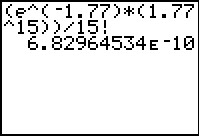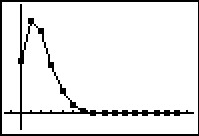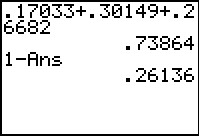••• ##### Device
• TI-83 Plus Family
• TI-84 Plus
• TI-84 Plus Silver Edition
•TI-84 Plus C Silver Edition
•TI-84 Plus CE
• ##### Report an Issue

Statistics: Is it Rare?
by Texas Instruments - Bell Ringer Lesson#### Overview

Students use the Poisson distribution to determine the probabilities for various numbers of hurricanes hitting the United States in a given year.

#### Key Steps

•In this activity, students will determine the probabilities of various numbers of hurricanes hitting the United States using a Poisson Distribution. They will accomplish this using the Home screen and the List Editor.

•Then students will explore how the graph of the Poisson Distribution changes when the average number of hurricanes changes by creating a connected scatter plot of the probabilities vs. number of hurricanes.

•At the end of the activity, students are introduced to finding cumulative probabilities. They are also given additional exercises to complete own their on, either in class or as homework.